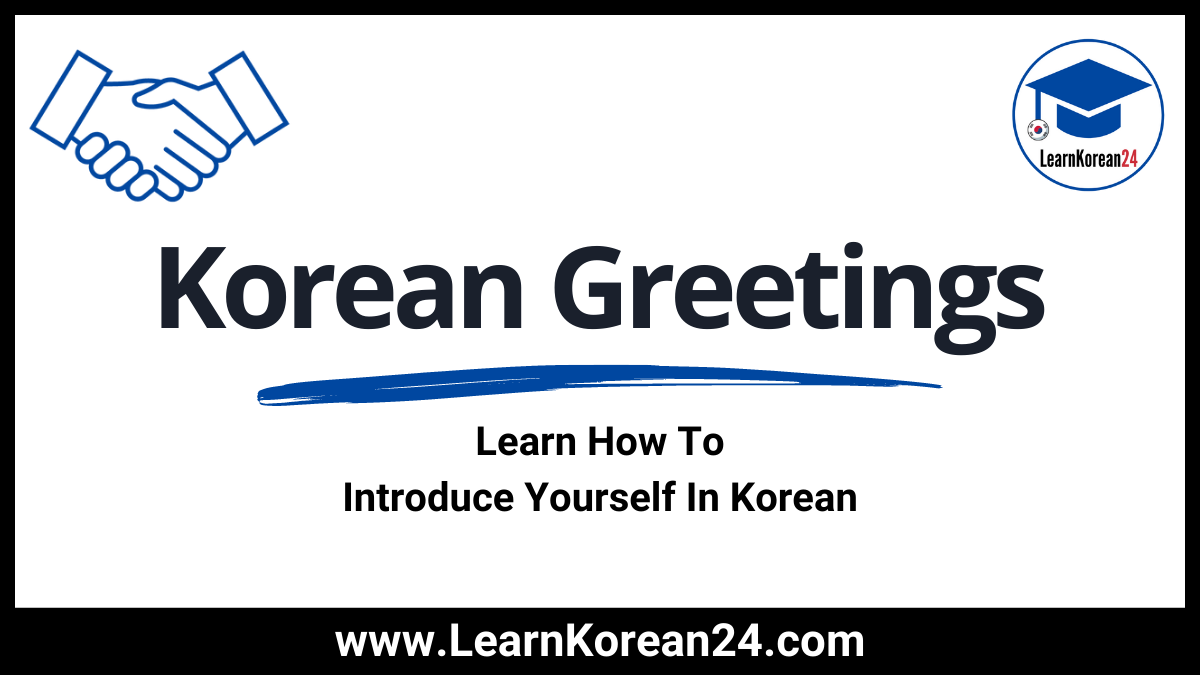# Korean Greetings | Learn How To Introduce Yourself In KoreanIn this lesson, you will learn Korean greetings and how to introduce yourself in Korean. First, let’s start by looking at the most common ways to greet someone in Korean.

## Korean Greetings (인사)

The word for ‘greeting’ in Korean is 인사 [in-sa]. Just like in English, there are several ways to greet someone in Korean, such as saying “Hello“, “Nice to meet you.“, etc. Here are the most common Korean greetings.

• 안녕. = Hi. (informal)
• 안녕하세요? = Hello. (polite)
• 안녕하십니까? = Hello. (formal)
• 반가워요. = Nice to meet you.
• 안녕히 가세요. = Goodbye. (used when the other person is leaving or both people are leaving.)
• 안녕히 계세요. = Goodbye. (used when you are leaving and the other person is staying.)
• 잘 가. = Goodbye. (informal)
• 어떻게 지내요? = How are you?
• 또 봐요. = See you again.
• 잘 자. = Good night. (informal)
• 안녕히 주무세요. = Good night. (formal)
• 실례합니다. = Excuse me.
• 밥 먹었니? = Did you eat? (informal)
• 식사하셨어요? = Did you eat? (formal)

## Korean Introductions (소개)

The word for ‘introduction’ in Korean is 소개 [so-gae]. Here is a list of common Korean introductions and phrases you can use when introducing yourself in Korean:

• 소개 = introduction
• 소개하다 = to introduce (verb)
• 자기소개 = self-introduction
• 제 소개를 하겠습니다. = Let me introduce myself.
• 이름 = name
• = last name
• 별명 = nickname
• 이름이 뭐예요? = What’s your name?
• 저는 수민 입니다. = I’m Sumin.
• 제 이름은 수민 입니다. = My name is Sumin.
• 어디에서 왔어요? = Where are you from?
• 미국에서 왔어요. = I’m from the U.S.A.
• 국적이 어떻게 돼요? = What is your nationality?
• 저는 미국 사람 이에요. = I’m American. (Literally, “I am an American person.”)